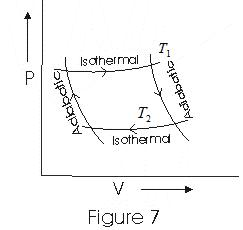# Carnot's Heat Engine

## Carnot's Heat Engine

• According to second law of thermodynamics, no heat engine can have 100% efficiency
• Carnot's heat engine is an idealized heat engine that has maximum possible efficiency consistent with the second law.
• Cycle through which working substance passed in Carnot's engine is known as Carnot's Cycle.
• Carnot's engine works between two temperatures
$T_1$ - temperature of hot reservoir
$T_2$ - temperature of cold reservoir
• In a Complete Carnot's Cycle system is taken from temperature T1 to T2 and then back from
temperature T2 to T1.
• We have taken ideal gas as the working substance of carnot engine.
• Fig below is an indicator digram for Carnot Cycle of an ideal gas(i) In step $b \rightarrow c$ isothermal expansion of gas taken place and thermodynamic variables of gas changes from (P1, V1,T1) to (P2,V2,T1)
• If Q1 is the amount of heat absorbed by working substance from the source and W1 the work done by the gas then Work done in an isothermal expansion
$Q_1 = W_1 = nRT_1 ln (\frac {V_2}{V_1})$ ---(1)
as process is isothermal.
(ii) Step $c \rightarrow d$ is an adiabatic expansion of gas from (P2, V2, T1) to (P3,V3,T2). Work done by gas in adiabatic expansion is given as
$W_2 =\frac { nR (T_1 - T_2)}{\gamma -1 }$ ---(2)
(iii)Step $d \rightarrow a$ is isothermal compression of gas from (P3,V3,T2) to (P4,V4,T2). Heat Q2 would be released by the gas to the at temperature T2
• Work done on the gas by the environment is
$W_3 = Q_2 = nRT_2 ln(\frac {V_3}{V_4})$ --(3)
(iv)Step $a \rightarrow b$ is adiabatic compression of gas from (P4, V4, T2) to (P1, V1, T1)
• Work done on the gas is
$W_4 =\frac {nR (T_1-T_2)}{(\gamma -1)}$ --(4)
• Now total work done in one complete cycle is
$W = W_1 + W_2 - W_3 - W_4$
$= nRT_1 ln (\frac {V_2}{V_1})-nRT_2 ln(\frac {V_3}{V_4})$ --(5)
as $W_2 = W_4$

## Efficiency of carnot engine

$\eta =\frac {W}{Q_1} = 1-(\frac {Q_2}{Q_1})$
$= 1-(\frac {T_2}{T_1}) \frac {ln(\frac {V_3}{V_4})}{ln( \frac {V_2}{V_1})}$ --(6)
Since points b and c lie on same isothermal
$\Rightarrow P_1V_1=P_2V_2$ --(7)
Also points c and d lie on same adiabatic
$\Rightarrow P_2(V_2)^{\gamma}=P_3(V_3)^{\gamma}$ ----(8)
Also points d and a lie on same isothermal and points a and b on same adiabatic thus,
$P_3V_3=P_4V_4$ ---(9)
$P_4(V_4)^{\gamma} =P_1(V_1)^{\gamma}$ ----- (9)
Multiplying all the above four eqns me get
$\frac {V_3}{V_4}= \frac {V_2}{V_1}$ ---(10)
Putting this in equation (5) we get
$\eta = 1-(\frac {T_2}{T_1})$ ---(11)
From above eqn we can draw following conclusions that efficiency of Carnot engine is
(i) independent of the nature of working substance
(ii) depend on temperature of source and sink
• A carnot refrigerator can be designed in the same manner, and we get the coefficient of performance as
$\alpha = \frac {T_2}{T_1 - T_2}$
This can also be expressed in Carnot engine efficiency as
$\alpha = \frac { 1 - \eta}{\eta}$

## Carnot Theorem

• Carnot Engine is a reversible engine.
• Carnot's theorem consists of two parts
(i) no engine working between two given temperatures can be more efficient than a reversible Carnot engine working between same source and sink.
(ii) all reversible engines working between same source and sink (same limits or temperature) have the same efficiency irrespective the working substance.

## Solved Examples

Question 1
Consider a Carnot's cycle operating between $T_1 = 500$ K and $T_2 = 300$ K producing 2 kJ of mechanical work per cycle. Find the heat transferred to the engine by the reservoirs?
Solution
Efficiency of Carnot's engine
$\eta = 1-(\frac {T_2}{T_1})$
$T_1 = 500$ K
$T_2 = 300$ K
$\eta = 1 - \frac {300}{500} =.4$
Also
$\eta = \frac {\text {Output work}}{\text {Heat Supplied}}= \frac {2000}{x}$
Now
$\frac {2000}{x} = .4$
x=5000J

Question 2
If the coefficient of performance of a refrigerator is 5 and operates at the room temperature (300 K), find the temperature inside the refrigerator?
Solution
We know that
$\alpha = \frac {T_2}{T_1 - T_2}$
$5 = \frac {T}{300 -T}$
or T=250 K